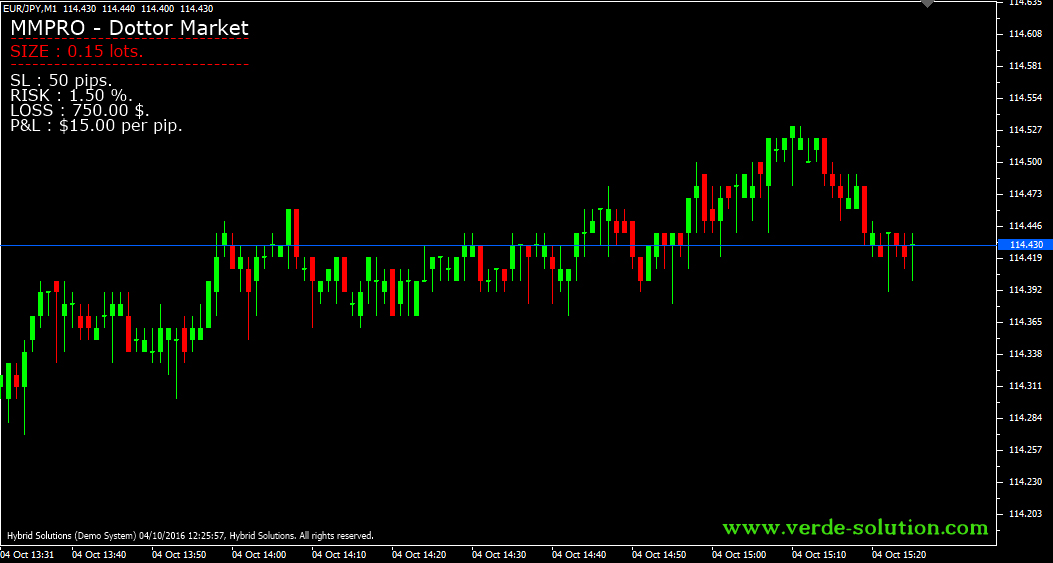# MMPRO Dottor Market

Free

Developed By: NeeduVerdeSolution

In stock

MMPRO Dottor Market indicator is a simple VertexFX client-side indicator script that is used by traders to calculate trade management and risk management parameters useful for trading.

SKU: 1366 Categories: ,MMPRO Dottor Market indicator is a simple VertexFX client-side indicator script that is used by traders to calculate trade management and risk management parameters useful for trading.

The user provides the stop-loss (STOP_LOSS_PIPS) and the risk per trade (RISK_PER_TRADE_PCT), then the indicator calculates the lot-size of the trade that must be opened, the absolute (dollar) amount of risk that the trade will incur and the profit or loss when the trade is favorable or unfavorable for every pip respective.
As mentioned earlier, it is used for reference to calculate lot-size for trading and for estimation of risk. It does not provide buy or sell signals.

The indicator displays the following information:
a) Size: The lot size to be traded for the specified stop-loss (STOP_LOSS_PIPS) and risk per trade (RISK_PER_TRADE_PCT) as calculated by the indicator.
b) Risk: The risk per trade (RISK_PER_TRADE_PCT) in percentage specified by the user.
c) Loss: The maximum loss (in dollars) calculated by the indicator based upon the risk per trade and the account equity.
d) P&L : The profit or loss (in dollars) calculated by the indicator for 1 pip movement in price of the instrument.

Parameters:

1) STOP_LOSS_PIPS – The stop-loss value of each trade in pips.
3) TICK_VALUE – The default value of a tick (in amount) for the current instrument. The indicator can query the value of a tick from the terminal if CALCULATE_TICK_VALUE is TRUE. If FALSE, then it uses this value for its calculations.
4) CALCULATE_TICK_VALUE – Specifies whether to calculate the value of a tick for the current instrument by querying the terminal. If this value is FALSE, the indicator uses the value specified by TICK_VALUE.
5) POINT_DEFAULT – The default value of a point for the current instrument. The indicator queries the value of a point from the terminal, but uses this value in case it cannot query the value from the terminal.